# Beer-Lambert’s Law Experiment

3468 words (14 pages) Essay

30th Jan 2018 Physics Reference this

Tags:

Disclaimer: This work has been submitted by a university student. This is not an example of the work produced by our Essay Writing Service. You can view samples of our professional work here.

Any opinions, findings, conclusions or recommendations expressed in this material are those of the authors and do not necessarily reflect the views of UKEssays.com.

Design Project on Beer- Lambert’s Law.

• Saswati Rakshit

Aim:

To learn and understand the basics and mathematical calculations of the following problem and write programs accordingly.

Problem 1: Suppose an outdoor multispectral image is captured by a camera with a path length of 1-3 microns. A part of the intensity is attenuated by the absorption of small particles in the atmosphere for that spectral range and let the scattering by the small particles for that spectral range is 0.

Find the total attenuation in the spectral range using Simpson 1/3 ­, trapezoidal and Euler integration methods and comment in your findings.

Scope/Application:

Beer Lambert’s law relates the attenuation of light to the properties of the material through which the light is passing. When light passes through a medium some amount of light is absorbed by the medium. For this absorption intensity of light reduces.

Beer Lambert’s law states that the quantity of light absorbed is directly proportional to the concentration of the substance and the path length of the light through the transmission medium

Beer Lambert’s law is used to find total attenuation of light when light passes through a medium(considering scattering is zero).It is also used to find the concentration of medium in chemical analysis, medium length in some application and absorbance of medium when necessary.

Introduction of Beer Lambert Law:

Lambert’s law is (Related to thickness/path length of medium)

When light passes through an absorbing medium its intensity decreases exponentially as the path length of the absorbing medium increases.

I = I0 e-k1 L ……………………………………….(i)

(where L is the length of medium and k1 is molar extinction/absorption co-efficient for the absorbing material)

Beer’s law is (Related to concentration of absorbing medium)

When light passes through medium(absorbing) its intensity decreases exponentially as the concentration of the absorbing medium increases.

I = I0e-k2C …………………………………..(ii)

(C concentration of medium and k2 is absorption co-efficient for the absorbing material)

Combining both Beer’s and Lambert’s law we get

I = I0 e-k3CL combining eqn (i) and (ii)

Where I0 = Incident light intensity

I = Transmitted Light intensity

C=concentration / volume

L= path length of medium

We consider an outdoor multispectral image is captured by a camera with a spectral range of 1-3 microns. A part of the intensity is attenuated by the absorption of small particles in the medium.so image will be attenuated. Beer Lambert’s law find the attenuation caused by absorption for that spectral range and let the scattering by the small particles for that spectral range is 0.

Objectives:

In a given path length 1 to 3 micron, we have considered a constant value of molar extinction/absorption co-efficient.and now we need to find the absorbance & total attenuation of the incident light using Beer’s Lambert Law. And then applying Simpson’s 1/3, Trapezoidal and Euler Integration in it compare the result.

System flow:

Implementation of the Beer Lambert’s law needs a proper mathematical understanding of the Beer Lambert’s law. Here I am showing how to set the equation using its mathematical basics

First we apply Beer Lambert’s Law for a medium which absorbs light in spectral range 1-3 micron. Considering no scattering we assume I0 is the incident light to the medium Air of attenuation coefficient 1.64at temperature 20oC. Here path length travelled by light is 1 to 3 micron.

According to Beer Lambert’s law light intensity is decreased if concentration & path length increase.

So we get the equation I=Io e-kcl = Io e-µl

Now as we know the path length l and attenuation coefficient µ, we calculate absorbance of the medium using eqn

Log10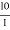= kcl (Where l is constant)= e kcl

But now for a spectral range 1 to 3 micron path length we need to formulate a new equation by integrating ranged from .001mm to .003 mm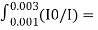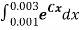[Here x is path length and c is attenuation coefficient]

Solving the above eqn we get total absorbance. Thus Beer Lambert’s law is successfully implemented in our problem.

Now we apply Simpson 1/3 rd, trapezoidal rule on Beer’s Lambert Law to find total attenuation.

Finally compare the result of Simpson’s 1/3 rd and Trapezoidal rule with the actual integration.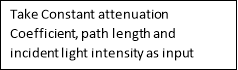Flow Diagram: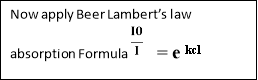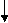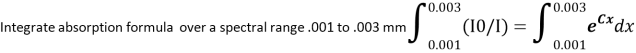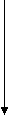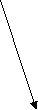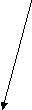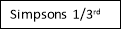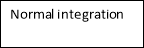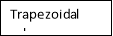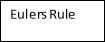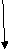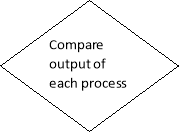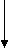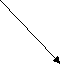Math:

Beer-Lambert Law Concept

Consider a light incident on a medium with area A and thickness dx and concentration of molecules C. Number of molecules illuminated by light of incident intensity Ix is CAdx.

Total effective area σCAdx. Probability of light being absorbed in thickness dx is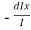=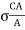dx [where dIx is the change in intensity across dx and σ is scattering coefficient]

So we can write,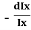=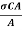dx ………… (i)

Now we integrate both sides of (i)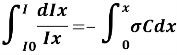ln (I) – ln(I0) = ln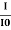= – σCx

I = I0e-σCx = I0 eµx ……………….(ii)

The co-efficient µ=σ C is the linear attenuation co-efficient. Here C=Absorbing co-efficient & σ = Scattering co-efficient.

The ibrightness of light decreases exponentially with depth in the medium.

So we can tell Beer-Lambert Law is also a function of( λ), i.e.

I (λ) = I0 (λ) e-µ(λ)x………………………(iii)

Calculation:

(Here we considered linear attenuation)

Given spectral range 0.001 mm to 0.003 mm

Let we consider Absorbing coefficient(C) of Transmission Medium (Air) = 1.64 at 20o C. So total absorption (A) of light is calculated by integrating in the spectral range,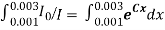[from eqn ii]

=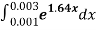———– (1)

=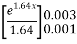= 0.002006571

Now we can easily calculate attenuated intensity of light (I).

Attenuation is the loss of light intensity over distance. The greater the distance, the lower is the intensity

Where I=I0 -Attenuation

Trapezoidal rule:-

We know, in case of multiple application of Trapezoidal rule, the formula is: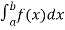=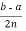[f(x0) + 2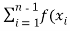) + f(xn)]

So, here applying the above formula for equation (1) we get: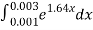= 0.0005[[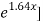0.001 + 2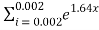+ [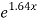] 0.003]

= 0.0005[1.001641346 + 2.00657077 + 1.004932123]

= 0.0005[4.013144239]

=0.002006572 (Ans.)

Here,

x0 = x0.001, xn = x0.003, b = 0.003, a = 0.001, n = 2,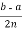= 0.0005.

Simpson’s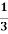rule:

We know, composite Simpson’s rule formula is written as:=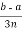[f(x0) + 4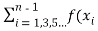) +2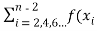) + f(xn)]

So, here applying the above formula for equation (1) we get:= 0.0003[[0.001 + 4+ [] 0.003]

= 0.0003[1.001641346 + 4.01314154 + 1.004932123]

= 0.001805914 (Ans.)

Here,= 0.0003.

Euler’s formula:

Here,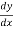=y(0.001) =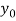= 0 (assumption)

y(0.003) =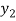and we have to find the value of equation (1) using Euler’s formula which is,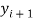=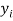+ f (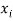,) h

let us choose h = 0.001

Step-1

i=0,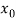= 0.001,= 0, h = 0.001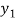=+ f (,) h

= 0 + f (0.001, 0) 0.001

= 0.001001641

Step-2

i=1,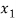= 0.002,= 0.001001641, h = 0.001=+ f (,) h

= 0.001001641 + f (0.001, 0.001001641) 0.001

= 0.0020049426

Thisis actually the value of the function at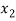i.e. at (+h) or (0.002+0.001) or 0.003.

So,=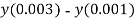= 0.0020049426-0

= 0.0020049426 (Ans.)

We find that the result of all above technique is almost same if we take approximation i.e.0.002.

CODES and OUTPUT:

Beer Lambert’s Law:

#include

#include

#include

int main()

{float absorbtion,m,l,u;

printf(“nEnter spectral range”);

scanf(“%f%f”,&l,&u);

printf(“nnenter the value of absorption cofficient”);

scanf(“%f”,&m);

absorbtion=(1/m)*(pow(2.718,(m*u))-pow(2.718,(m*l)));

printf(“nnTotal absorption is %f: “,absorbtion);

getch();

}

Output: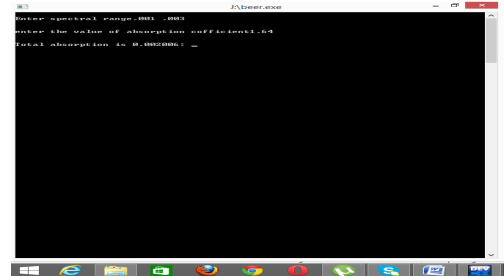Simpson 1/3rd rule:

#include

#include

#include

void main()

{float x,y,Total=0,h,t;

int i,n,j,k=0;

printf(“nhow many values you will enter: “);

scanf(“%d”,&n);

for(i=0; i

{ printf(“nn x%d: “,i);

scanf(“%f”,&x[i]);

printf(“nn f(x%d): “,i);

scanf(“%f”,&y[i]); }

h=x-x;

n=n-1;

Total = Total + y;

for(i=1;i

{ if(k==0) { Total = Total + 4 * y[i];

k=1 }

else { Total = Total + 2 * y[i];

k=0; } }

Total = Total + y[i];

Total = Total * (h/3);

printf(“nn I = %f “, Total);

getch();}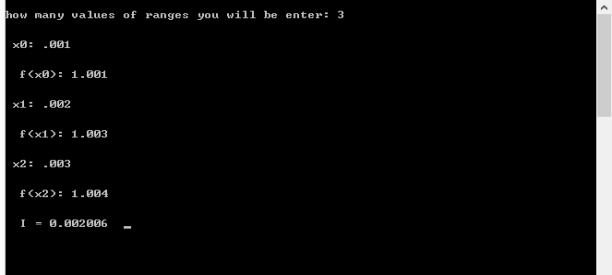Trapezoidal rule:

#include

#include

#include

int main(){

float x,y, Total =0,h;

int i,n,j,k=0;

float fact(int);

printf(“nhow many values of ranges you will be enter: “);

scanf(“%d”,&n);

for(i=0; i

{printf(“nn x%d: “,i);

scanf(“%f”,&x[i]);

printf(“nn f(x%d): “,i);

scanf(“%f”,&y[i]); }

h=x-x;

n=n-1;

for(i=0;i

if(k==0) { Total = Total + y[i];

k=1; }else

Total = Total + 2 * y[i];}

Total = Total + y[i];

Total = Total * (h/2);

printf(“nn I = %f “, Total);

getch();}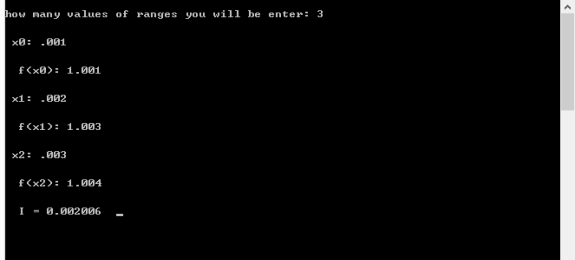Future Work & Scope:

This Beer Lambert’s law can be used in image processing application where atmospheric condition is poor to find the attenuation of light and image by absorption of light.

Implementing Euler Method.

References:

1. Weisstein, Eric W. “Simpson’s Rule.” From MathWorld–A Wolfram Web Resource. http://mathworld.wolfram.com/SimpsonsRule.html. (Accessed on 26.04.2015)
2. Basics of Trapezoidal and Simpson Rules, www.math.umd.edu/~jmr/141/Simpson.pdf.
3. Lal, A. K., Simpson’s Rule, 2007, http://numericalmethods.eng.usf.edu.(Accessed on 20.04.2015)
4. http://numericalmethods.eng.usf.edu. (Accessed on 19.03.2015)
5. Garrett, P., Absorption and Transmission of light and the Beer-Lambert Law, Lecture 21, 2006, www.physics.uoguelph.ca/~pgarrett/Teaching.html. (Accessed on 26.04.2015)
6. Mudakavi, J. R., Modern Instrumental Methods of Analysis, Lecture – 07, Ultraviolet and Visible Spectrophotometry – 3 Theoretical Aspects, http://nptel.ac.in/courses/103108100/7 (Accessed on 26.04.2015).
7. www.chemwiki.ucdavis.edu. (Accessed on 19.03.2015)

## Related Services

View all

### DMCA / Removal Request

If you are the original writer of this essay and no longer wish to have your work published on the UKDiss.com website then please:

Related Services